# Second Challenge Problem 3

Problem 3 Summary

Determine the heat of mixing for 2 binary systems at 4 equally spaced compositions and 2 temperatures (a total of 16 state points). The first mixture is of a liquid amine and a hydrocarbon (n-butylamine [CAS # 109-73-9] and n-heptane [CAS #142-82-5]) and the second is of the same liquid amine and water (n-butylamine [CAS # 109-73-9] and water [CAS # 7732-18-5]).

n-butylamine

Hill formula: C4H11N

CAS # 109-73-9

Other names:
1-Aminobutane
1-Butanamine
1-Butylamine
Butylamine
Butylamine, n
Monobutylamine
Mono-n-butylamine
n-C4H9NH2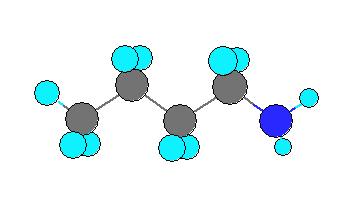n-heptane

Hill formula: C7H16

CAS # 142-82-5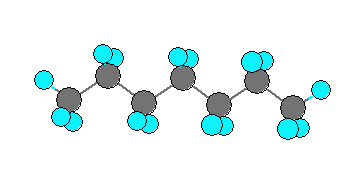water

Hill formula: H2O

CAS # 7732-18-5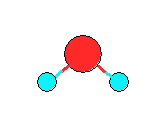Introduction

Real binary mixture behavior is frequently described through the excess properties . For liquids far away from the critical point, properties are not strongly influenced by pressure. The properties of primary interest are GE, the excess Gibbs energy, and its temperature derivatives, particularly HE, the excess enthalpy of mixing or short heat of mixing: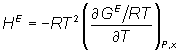(1)

The logarithm of the activity coefficient &gamma;i is the partial molar property with respect to the dimensionless excess Gibbs energy: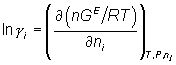(2)

One the premier challenges for modeling thermal separation processes (e.g., absorption, distillation, extraction, etc.) is the ability to describe activity coefficients of the components of interest over the whole concentration and temperature range of interest with sufficient accuracy.

For many years chemical engineers and chemists involved with chemical process simulations have relied on well-known local composition GE-models (e.g., UNIQUAC, NRTL, Wilson) to model cases where non-ideal behavior in the liquid phase plays an important role. The parameters of those GE-models require experimental (or if appropriate, predicted) phase equilibrium data. There are many types of phase equilibrium data that can be used for regression, but isothermal vapor-liquid equilibria are probably one of the most common types that is being used in the chemical industry.

One of the inherent problems with using the aforementioned GE-models is that their built-in capability to extrapolate with high accuracy outside the range of the fitted data is usually quite unreliable. The common way to solve this problem is to use phase equilibrium data for the parameter regression that cover the whole range of temperatures of interest. Introducing an empirical function into the GE-model that allows the parameters to be temperature dependent usually solves this problem. In practical terms this means that more experimental data needs to be made available. Common practice is to use vapor-liquid equilibria (VLE) data at 2 or 3 temperatures for the parameters regression or sometimes by combining VLE and heat of mixing data. There are practical consequences from the selection of which phase equilibrium data should be chosen for parameter regression, but those consequences are less importance for this competition.

Equations (1) and (2) show that if it is desirable to describe activity coefficients as function of temperature with high accuracy any GE-model used needs to be able to describe the heat of mixing properly as well. As a consequence, erroneous activity coefficients may result in erroneous K-factors or distribution coefficients which will in turn lead to a less than optimal design of the separation equipment. The result could be over-design or worse under-design of the equipment making the targeted separation more expensive or even impossible than with an optimal design.

In the first competition one of the problem sets given was to predict the total pressure of a binary mixture as function of composition at two constant temperatures. A subset required the prediction of azeotropic pressures and compositions of a different binary.

Problem Definition

Part 3-1: Determine the heat of mixing of n-butylamine [CAS # 109-73-9] and n-heptane [CAS # 142-82-5] at n-butylamine mole fractions of 0.2, 0.4, 0.6, and 0.8 and temperatures of 298 and 348 K and a pressure of 101.325 kPa (a total of 8 state points). Also specify the uncertainty in the reported values.

Part 3-2: Determine the heat of mixing of n-butylamine [CAS # 109-73-9] and water [CAS # 7732-18-5] at n-butylamine mole fractions of 0.2, 0.4, 0.6, and 0.8 and temperatures of 298 and 348 K and a pressure of 101.325 kPa (a total of 8 state points). Also specify the uncertainty in the reported values.

Note: The pressure of 101.325 kPa specified in parts 3-1 and 3-2 is a suggestion.  The actual pressures used in the experimental measurements are likely to be slightly different.  Likewise, it is permissible for simulators to use other values of pressure in their calculations for this problem.  Since the heat of mixing has no significant pressure-dependence within a reasonable range of pressure around 101.325 kPa, small changes in the pressure will not affect the results but may have practical experimental or computational advantages and, therefore, are permissible.

Problem Requirements and Restrictions

1. Any force-field parameters (published or unpublished) may be used as long as they do not violate restriction 3 and 4 below and are reported in the manuscript submitted to the contest committee. Any force-field parameters published in a peer-reviewed journal by the date of the Contest announcement may be used, regardless of what experimental data were used to derive them..

2. Any public pure component data published by the date of the Contest announcement may be used by contestants for method validation.

3. Any public mixture data published by the date of the Contest announcement may be used by contestants for method validation, with exception of any mixtures (be it binary or higher order) involving any of the binary systems of the contest.

4. The same amine force-field parameters (or whatever parameters are required by your simulation method) must be used in both problems.

5. If heat of mixing cannot be obtained directly by the simulation method used, it is allowed to use thermodynamic relations (for example, equations 1 or 2) to numerically calculate the heat of mixing.

Judging Criteria

1. A maximum of 100 points can be achieved for this problem set.

2. 20% (or 20 points) of the score is based on predicting the correct trend with temperature (or the slope &Delta;HE/&Delta;T) for each system. In other words does the maximum value of HE increase, decrease, or stay nearly constant with temperature (Is the slope &Delta;HEE/&Delta;T positive, negative, or nearly constant?). Note: The maximum value of HE as a function of mole fraction might not coincide with the selected state points of the competition nor with equal mole fraction. It is allowed to use a fitting function (for example, the Redlich-Kister expansion ) to determine the maximum of HE for each temperature. For the purposes of determining the score, nearly constant is defined as the maximum HE values at the two temperatures are 10% or less apart.

3. 80% (or 80 points) of the score is based on a quantitative comparison of the predicted HE values with the experimental values. Deviations are calculated as the relative difference between experimental and calculated. No credit is given for a state point if it is not calculated or deviates by > 100%. 0% deviation will be assigned if a calculated point is within the experimental error.

The weighting factors for problems 3-1 and 3-2 are 0.4 and 0.6 respectively to reflect that the 2nd problem has a higher importance from the committee’s point of view.